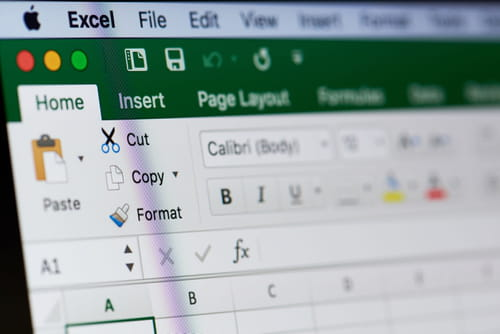24
Thanks

A few words of thanks would be greatly appreciated.

# Transfer Data Between Excel Spreadsheets

This article will teach you how to transfer data from one spreadsheet to another in Microsoft Excel if your copy and paste function is not working. The two methods presented will use a quick formula that you can apply to data present in the same Microsoft Excel file.## Transfer Data from One Spreadsheet to Another

For each example, consider that we have two sheets: Sheet 1 and Sheet 2 and we would like to transfer from cell A1 of Sheet 1 to cell B1 of Sheet 2.

### Using the + Symbol in Excel

Start by selecting the target cell (in our case B1 of Sheet 2) and typing in the + symbol.

Next, right-click on the Sheet 1 label button to go back to your data. Select cell A1 and then press Enter.

Your data will be automatically copied into cell B1.

If you performed the operation correctly, then upon selecting cell A1, you should have the following formula displayed in the formula bar: +Sheet1!A1.

### Using the +Sheet(X)!((XY) Formula

The second method will make use of the +Sheet(X)!(XY) formula.

Select the cell in which you would like to swap the data and type

`+Sheet(X)!(XY)`

into the formula bar.

Using the conditions above, the formula +Sheet2!B21 will copy data from cell B21 of Sheet 2.

N.B. "X" stands for "sheet label;" and "XY" stands for the targeted cell coordinates.

Image: © Dzmitry Kliapitski - Shutterstock.com

24
Thanks

A few words of thanks would be greatly appreciated.